In :
%pylab inline

Welcome to pylab, a matplotlib-based Python environment [backend: module://IPython.zmq.pylab.backend_inline].

In :
import numpy as np
from pandas import *
import matplotlib.pyplot as plt
import os
import statsmodels.api as sm
from statsmodels.nonparametric.kde import KDE
from statsmodels.nonparametric.smoothers_lowess import lowess
from statsmodels.formula.api import logit, glm


# Numeric summaries of height and weight data¶

First, let's import the data and take a look at the first few observations to get a sense of the units. Height appears to be in inches, Weight in pounds. And Gender is a string coded Male or Female.

In :
heights_weights = read_table('data/01_heights_weights_genders.csv',
sep = ',', header = 0)
print heights_weights['Gender'].value_counts()

  Gender     Height      Weight
0   Male  73.847017  241.893563
1   Male  68.781904  162.310473
2   Male  74.110105  212.740856
3   Male  71.730978  220.042470
4   Male  69.881796  206.349801
Male      5000
Female    5000


A more detailed summary of the Height variable.

In :
heights = heights_weights['Height']
heights.describe()

Out:
count    10000.000000
mean        66.367560
std          3.847528
min         54.263133
25%         63.505620
50%         66.318070
75%         69.174262
max         78.998742

## Means, medians, and modes¶

Pretty basic stuff. We'll just make some home-rolled versions of basic mean and median functions, just to follow the text (p. 38).

In :
def my_mean(x):
return float(np.sum(x)) / len(x)

In :
def my_median(x):
'''
Compute the median of a series x.
'''

# Get a sorted copy of the values in the series (need to call values
# otherwise integer indexing messes things up.)
sorted_x = np.sort(x.values)
if len(x) % 2 == 0:
indices = [0.5 * len(x) - 1, 0.5 * len(x)]
return np.mean(sorted_x[indices])
else:
# Ceil(x) - 1 = Floor(x), but this is to make clear that the -1 is to
# account for 0-based counting.
index = ceil(0.5 * len(x)) - 1
return sorted_x.ix[index]


Check my_mean and my_median against built-ins

In :
my_mean(heights) - heights.mean()

Out:
0.0
In :
my_median(heights) - heights.median()

Out:
0.0

## Quantiles¶

See p. 40 of the book.

In :
print 'Range of heights (in.): ', heights.min(), heights.max()

Range of heights (in.):  54.2631333251 78.9987423464

In :
# Range = max - min. Note: np.ptp(heights.values) will do the same thing.
# HT Nathaniel Smith

In :
def my_range(s):
'''
Difference between the max and min of an array or Series
'''
return s.max() - s.min()

print 'Range width of heights (in.) (my_range()): ', my_range(heights)
print 'Range width of heights (in.) (np.ptp()): ',np.ptp(heights)

Range width of heights (in.) (my_range()):  24.7356090213
Range width of heights (in.) (np.ptp()):  24.7356090213


Pandas doesn't seem to provide multiple arbitrary quantiles. But (1) the standard ones are available via .describe() and (2) creating one is simple.

To get a single quantile of a pandas Series:

In :
heights.quantile(.5)

Out:
66.318070081784654

A function to get an arbitrary set of quantiles of a pandas Series. Give quartiles by default.

In :
def my_quantiles(s, prob = (0.0, 0.25, 0.5, 1.0)):
'''
Calculate quantiles of a series.

Parameters:
-----------
s : a pandas Series
prob : a tuple (or other iterable) of probabilities at
which to compute quantiles. Must be an iterable,
even for a single probability (e.g. prob = (0.50)
not prob = 0.50).

Returns:
--------
A pandas series with the probabilities as an index.
'''
q = [s.quantile(p) for p in prob]
return Series(q, index = prob)

# With the default prob argument
my_quantiles(heights)

Out:
0.00    54.263133
0.25    63.505620
0.50    66.318070
1.00    78.998742

For another example, deciles:

In :
my_quantiles(heights, prob = arange(0, 1.1, 0.1))

Out:
0.0    54.263133
0.1    61.412701
0.2    62.859007
0.3    64.072407
0.4    65.194221
0.5    66.318070
0.6    67.435374
0.7    68.558072
0.8    69.811620
0.9    71.472149
1.0    78.998742

## Standard deviation and variances¶

Again, some home-rolled functions for illustration, checked against the numpy versions.

In :
def my_var(x):
return np.sum((x - x.mean())**2) / (len(x) - 1)

In :
my_var(heights) - heights.var()

Out:
-2.2106760866336117e-11
In :
def my_sd(x):
return np.sqrt(my_var(x))

In :
my_sd(heights) - heights.std()

Out:
-2.8728130985200551e-12

# Exploratory Data Visualization¶

## Histograms¶

We'll plot some historgrams, experimenting with bin width. Matplotlib's hist function lets you specify the number of bins, or give an explicit set of bins, but you can't specify just the bin width. So to match the book, we'll create explicit bins with a given width using np.arange.

In :
# 1-inch bins
bins1 = np.arange(heights.min(), heights.max(), 1.)
heights.hist(bins = bins1, fc = 'steelblue')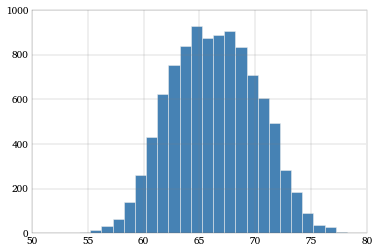In :
# 5-inch bins
bins5 = np.arange(heights.min(), heights.max(), 5.)
heights.hist(bins = bins5, fc = 'steelblue')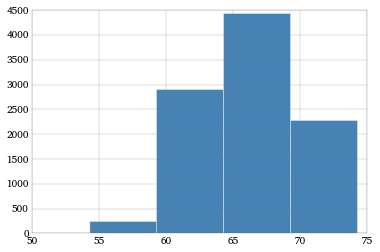In :
# 0.001-inch bins
bins001 = np.arange(heights.min(), heights.max(), .001)
heights.hist(bins = bins001, fc = 'steelblue')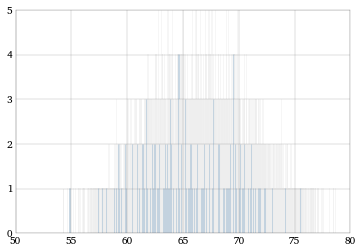# Kernel density estimators with statsmodels¶

Similar most models in the statsmodels API, we'll compute the KDE by calling it on the data, which produces a KDE object. Then we'll call the fit method on that object, which will give us a number of attributes, including the density attribute, which is the KDE result we're looking for.

In :
heights_kde = KDE(heights)
heights_kde.fit()


Plot the density of the heights. Sort inside the plotting so the lines connect nicely.

In :
fig = plt.figure()
plt.plot(heights_kde.support, heights_kde.density)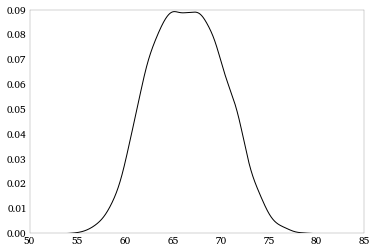We'll pull out male and female heights as separate arrays over which we'll compute densities

In :
heights_m = heights[heights_weights['Gender'] == 'Male'].values
heights_f = heights[heights_weights['Gender'] == 'Female'].values
heights_m_kde = KDE(heights_m)
heights_f_kde = KDE(heights_f)
heights_m_kde.fit()
heights_f_kde.fit()

fig = plt.figure()
plt.plot(heights_m_kde.support, heights_m_kde.density, label = 'Male')
plt.plot(heights_f_kde.support, heights_f_kde.density, label = 'Female')
plt.legend()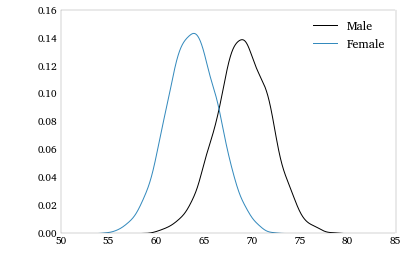And we'll repeat with weights.

In :
weights_m = heights_weights[heights_weights['Gender'] == 'Male']['Weight'].values
weights_f = heights_weights[heights_weights['Gender'] == 'Female']['Weight'].values
weights_m_kde = KDE(weights_m)
weights_f_kde = KDE(weights_f)
weights_m_kde.fit()
weights_f_kde.fit()

fig = plt.figure()
plt.plot(weights_m_kde.support, weights_f_kde.density, label = 'Male')
plt.plot(weights_f_kde.support, weights_f_kde.density, label = 'Female')
plt.legend()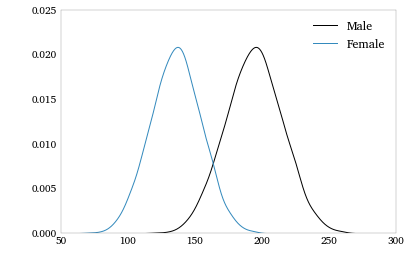Put these in a stacked plot, like in the book.

In :
fig, axes = plt.subplots(nrows = 2, ncols = 1, sharex = True, figsize = (9, 6))
axes.plot(weights_f_kde.support, weights_f_kde.density, label = 'Female')
axes.xaxis.tick_top()
axes.legend()
axes.plot(weights_m_kde.support, weights_f_kde.density, label = 'Male')
axes.legend()
plt.xlabel('Weight (lbs.)')

Out:
<matplotlib.text.Text at 0x10920cc90>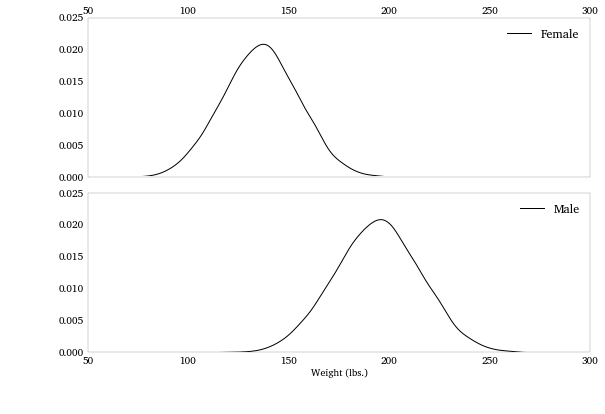# Scatterplots and lowess smoothing¶

Pull weight (for both sexes) out as a separate array first, like we did with height above, and scatter plot them.

In :
weights = heights_weights['Weight']
plt.plot(heights, weights, '.k', mew = 0, alpha = .1)

Out:
[<matplotlib.lines.Line2D at 0x125855e10>]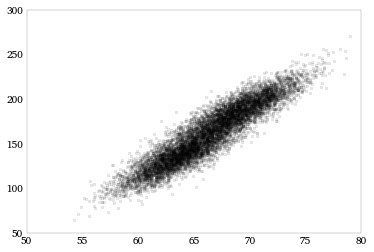Compute the lowess smoother. The lowess function in statsmodels is pretty slow. (For a discussion on why, and a faster version, see: this blog post.)

In :
lowess_line = lowess(weights, heights)

In :
plt.figure(figsize = (10, 7))
plt.plot(heights, weights, '.', mfc = 'steelblue', mew=0, alpha = .25)
plt.plot(lowess_line[:,0], lowess_line[:, 1],  '-', lw = 2,
color = '#461B7E', label = "Lowess fit")
plt.legend(loc = "upper left")

Out:
<matplotlib.legend.Legend at 0x109236b50>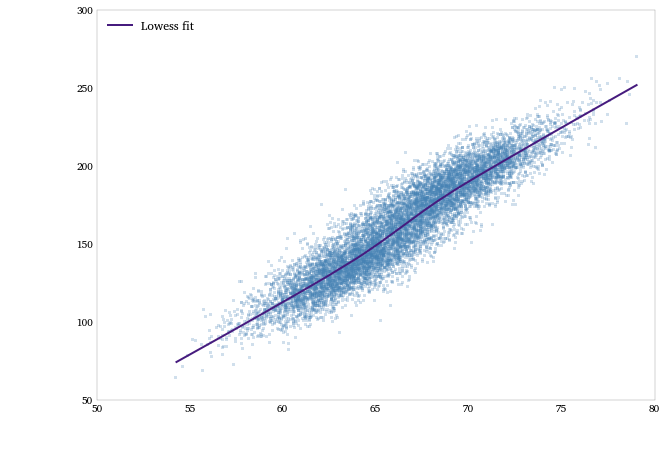# Logistic regression of sex on height and weight¶

For logistic (and GLM as we'll see below) regression we can use statsmodels's formula interface (using patsy).

To do this, lets first make a binary variable, Male, which equal 1 if Gender is Male and 0 otherwise. There are a number of ways to do this, but for fun, and to be explicit, I'll use the map method in pandas.

In :
heights_weights['Male'] = heights_weights['Gender'].map({'Male': 1, 'Female': 0})
male_logit = logit(formula = 'Male ~ Height + Weight', df = heights_weights).fit()
print male_logit.summary()

Optimization terminated successfully.
Current function value: 2091.297971
Iterations 8
Logit Regression Results
==============================================================================
Dep. Variable:                   Male   No. Observations:                10000
Model:                          Logit   Df Residuals:                     9997
Method:                           MLE   Df Model:                            2
Date:                Thu, 20 Dec 2012   Pseudo R-squ.:                  0.6983
Time:                        14:41:33   Log-Likelihood:                -2091.3
converged:                       True   LL-Null:                       -6931.5
LLR p-value:                     0.000
==============================================================================
coef    std err          z      P>|z|      [95.0% Conf. Int.]
------------------------------------------------------------------------------
Intercept      0.6925      1.328      0.521      0.602        -1.911     3.296
Height        -0.4926      0.029    -17.013      0.000        -0.549    -0.436
Weight         0.1983      0.005     38.663      0.000         0.188     0.208
==============================================================================


We can do the same thing with the glm function, if we specify the binomial family and logit link. This is typically how I'd go about this in R.

In :
male_glm_logit = glm('Male ~ Height + Weight', df = heights_weights,
print male_glm_logit.summary()

                 Generalized Linear Model Regression Results
==============================================================================
Dep. Variable:                   Male   No. Observations:                10000
Model:                            GLM   Df Residuals:                     9997
Model Family:                Binomial   Df Model:                            2
Method:                          IRLS   Log-Likelihood:                -2091.3
Date:                Thu, 20 Dec 2012   Deviance:                       4182.6
Time:                        14:41:37   Pearson chi2:                 9.72e+03
No. Iterations:                     8
==============================================================================
coef    std err          t      P>|t|      [95.0% Conf. Int.]
------------------------------------------------------------------------------
Intercept      0.6925      1.328      0.521      0.602        -1.911     3.296
Height        -0.4926      0.029    -17.013      0.000        -0.549    -0.436
Weight         0.1983      0.005     38.663      0.000         0.188     0.208
==============================================================================


We can use the logit parameters to draw a separating line in the (height, weight)-space. The line will separate the space into predicted-male and predicted-female regions.

The calculations below transform the logit model parameters into the slope and intercept of the separating line.

In :
logit_pars = male_logit.params
intercept = -logit_pars['Intercept'] / logit_pars['Weight']
slope =  -logit_pars['Height'] / logit_pars['Weight']


Color coding the points by sex in (height-weight)-space, we can see how well the logit classifies sexes.

In :
fig = plt.figure(figsize = (10, 8))
# Women points (coral)
plt.plot(heights_f, weights_f, '.', label = 'Female',
mfc = 'None', mec='coral', alpha = .4)
# Men points (blue)
plt.plot(heights_m, weights_m, '.', label = 'Male',
mfc = 'None', mec='steelblue', alpha = .4)
# The separating line
plt.plot(array([50, 80]), intercept + slope * array([50, 80]),
'-', color = '#461B7E')
plt.xlabel('Height (in.)')
plt.ylabel('Weight (lbs.)')
plt.legend(loc='upper left')

Out:
<matplotlib.legend.Legend at 0x10b11e790>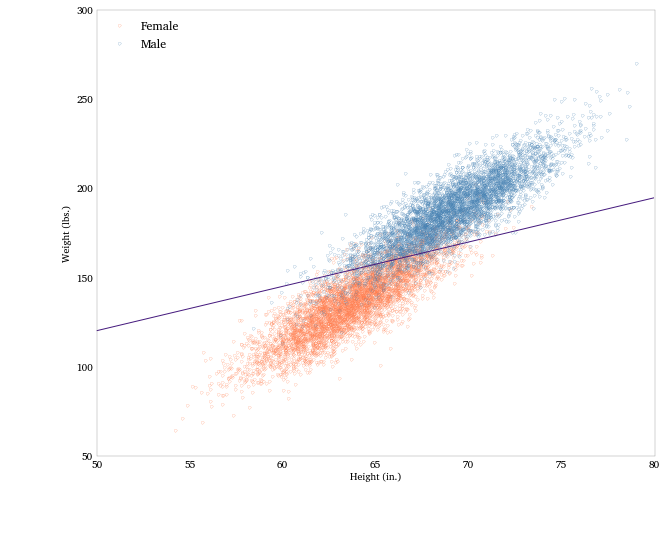In [ ]: